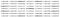# Deblurring Images for OSINT and more — Part 1

During intelligence operations, we often come across images that may contain important information but they are too blurred, noisy or just unclear to make sense out of. In this article, I am going to talk about some techniques that we can use to retrieve information from blurred images.

## How do filters work?

Every image is made up of tiny dots, known as Pixels. Each pixel has a value which produces the color it has. Different picture formats require the pixels to hold a different kind of values. For example, grayscale images are “black and white” and each pixel in them holds a value ranging from 0 to 255 where 0 is pure black and 255 is pure white while a pixel of an RGB image contains three values, Red, Blue, and Green e.g.(0, 124, 255). All colors can be formed using these three primary colors. Interesting. isn’t it?
So the photo filters just modify these values using mathematical operations. Let’s consider a 6x6 grayscale image with pixels having values ranging from 0 to 255

We will apply a filter on it using something we call a Kernel. Both the image and the kernel are matrices.
Let’s use this 3x3 Kernel

We will place this kernel over our image like this

Let’s multiply values of the kernel and the overlayed values of the image

`(94 x 0) + (178 x 2) + (124 x 0) + (23 x 2) + (94 x 4) + (135 x 2) + (153 x 0) + (120 x 2) + (140 x 0)Let's do some maths!0 + 356 + 0 + 46 + 376 + 270 + 0 + 240 + 0Doing some more maths gives as 1288Now let's divide 1288 by the sum of values of the kernel i.e. 121288 / 12 = 107`

Now, this is the new value of the pixel in the center (the one having 94 value).
So yeah...

We keep moving our kernel all over the image

We keep doing this to get the new values for the pixel in the center of the kernel. No, I am not going to calculate the value of each pixel now but you get the idea.

So this is how filters work. The values and dimension of the kernel determine what the output image will be. For example, the following kernel will Sharpen the image

` 0  -1   0-1   5  -1 0  -1   0`

But we are interested in blur filters and the most common type of blur is Gaussian blur which is done using Gaussian function and its kernel looks something like this:Source: WikiPedia

Notice how the values are being normalized and how the values start decreasing with distance from the center? That’s what reduces the details in images and makes them look blurry.

## Reversing Blur

Quick question, I divided 10 with x and got 2, what’s the value of x? 5, right? We had 2 inputs i.e. 10 and x, an operator i.e. division and the result i.e., 2. By using this information we were able to find the value of x. Can we undo the Gaussian blur same way? Yes, to an extent we can.

The operation that we performed on our image earlier is called convolution and all filters work on the same principle. To unblur the image we will use self explanatory operation, deconvolution.

Let’s take this image for example

Let’s use Gaussian blur of radius 3 on this

If you want to try it yourself, download GIMP and install a plugin names G’MIC. After installing, go to Filters>G’MIC>Details>Sharpen (Deblur) to access the concerned tool.

Anyway, using deconvolution with appropriate values produces this result which is readable

For better comparison, check this out

Maths is cool, isn’t it?

Well, that’s all for now. We will talk about more techniques in the next article in this series.

Further reading:

I make stuff, I break stuff and I make stuff that breaks stuff.

## More from Somdev Sangwan

I make stuff, I break stuff and I make stuff that breaks stuff.